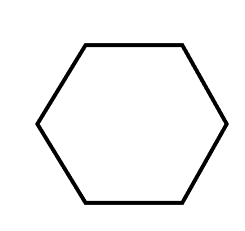Courses
Courses for Kids
Free study material
Offline Centres
MoreLast updated date: 26th Nov 2023
Total views: 281.1k
Views today: 8.81k

# Each angle of a regular hexagon is $\_\_\_\_$.Verified
281.1k+ views
Hint: Before solving this question we should know what a hexagon is. A hexagon is a type of polygon which has $6$ sides and $6$ vertices. We know that there are two types of hexagon- (a) Regular hexagon and (b) Irregular hexagon. A regular hexagon has all angles of the same measures and all sides of the same length. We can say that a regular hexagon is equilateral as well as equiangular.

Complete step by step solution:
Here we have to find the measure of each angle of a regular hexagon.
Let us first draw the image of a regular hexagon.Now we know that the sum of the internal angles of a regular hexagon is always ${720^ \circ }$.
We also know that all the angles and sides of a regular hexagon are equal, so let us assume that the measure of each angle is $x$.
Now we can write $x + x + x + x + x + x = 720$ (Sum of the interior angles of a hexagon).
On solving we have $6x = 720$, so it gives us $x = \dfrac{{720}}{6} = 120$.
Hence the measure of each angle of a regular hexagon is ${120^ \circ }$.

Note:
We should note that hexagon is also further classified into Convex or concave. If a hexagon is convex then none of its interior angle would be greater than ${180^ \circ }$ and if the hexagon is concave then the one or more of its interior angles is greater than ${180^ \circ }$. We should have the clear concept of different types of polygons and their properties before solving this kind of question.